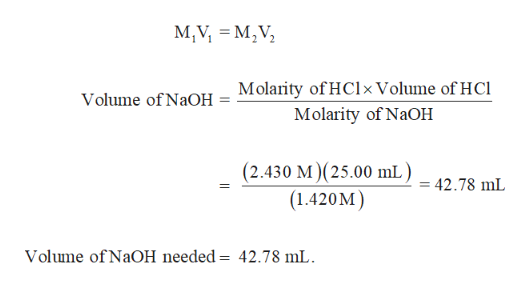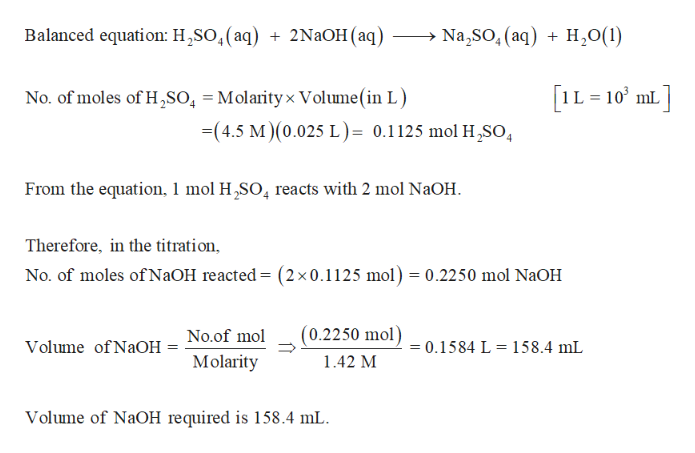11. Calculate the volume in mL of a 1.420 M NaOH solution required to titrate the following solutions: a. 25.00 mL of a 2.430 M HCl solutionb. 25.00 mL of a 4.500 M H2SO4 solutionc. 25.00 mL of a 1.500 M H3PO4 solution

Question

11. Calculate the volume in mL of a 1.420 M NaOH solution required to titrate the following solutions:

a. 25.00 mL of a 2.430 M HCl solution
b. 25.00 mL of a 4.500 M H2SO4 solution
c. 25.00 mL of a 1.500 M H3PO4 solution

Step 1

(a)

Volume of NaCl needed to titrate 25.00 mL of 2.430 M HCl solution is determined as follows,help_outlineImage TranscriptioncloseM,V M,V Molarity of HClxVolume of HCl Molarity of NaOH Volume of NaOH (2.430 M) (25.00 mL) = 42.78 mL (1.420M) Volume of NaOH needed = 42.78 mL fullscreen
Step 2

(b)

H2SO4 is a diprotic acid. Volume of NaCl needed to titrate 25.00 mL of 4.500 M H...help_outlineImage TranscriptioncloseBalanced equation: H2SO,(aq) Na,SO, (aq)H0(1) 2NaOH (aq) IL 10 mL No. of moles of H2SO, = Molarityx Volume (in L) -(4.5 M)(0.025 L)= 0.1125 mol H2SO 4 From the equation, 1 mol H,SO4 reacts with 2 mol NaOH Therefore, in the titration No. of moles of N2OH reacted = (2x0.1125 mol) = 0.2250 mol N2OH (0.2250 mol) No.of mol 0.1584 L= 158.4 mL Volume of NaOH Molarity 1.42 M Volume of NaOH required is 158.4 mL fullscreen

Want to see the full answer?

See Solution

Want to see this answer and more?

Our solutions are written by experts, many with advanced degrees, and available 24/7

See Solution
Tagged in

Quantitative analysis